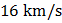# At some point the gravitational potential and also the gravitational field due to earth is zero. The speed is a) On earth’s surface b) Below earth’s surface c) At a heightfrom earth’s surface (radius of the earth) d) At infinity

## Question ID - 55026 :- At some point the gravitational potential and also the gravitational field due to earth is zero. The speed is a) On earth’s surface b) Below earth’s surface c) At a heightfrom earth’s surface (radius of the earth) d) At infinity

3537

 (d)andandatA satellite is to revolve round the earth in a circle of radius. The speed at which this satellite be projected into an orbit, will be a)b)c)d)